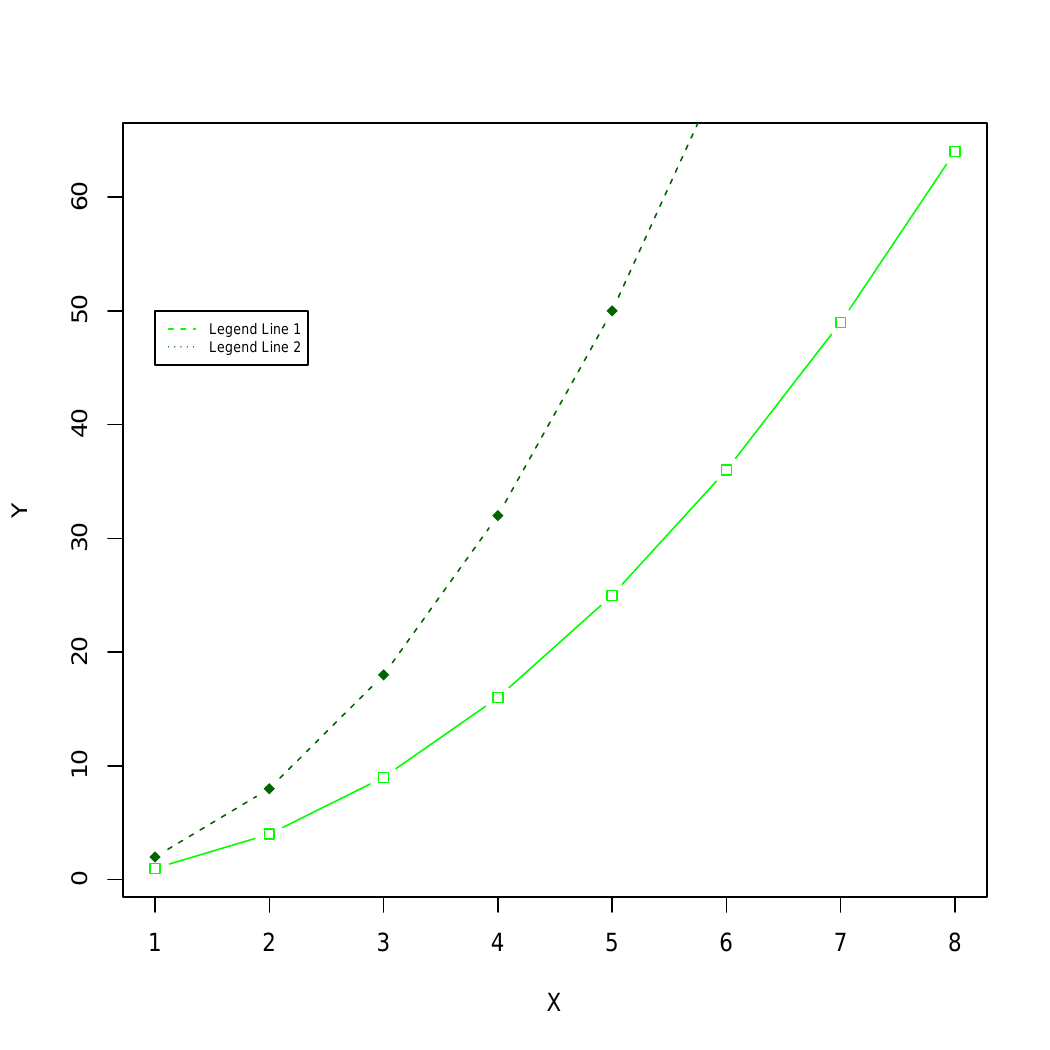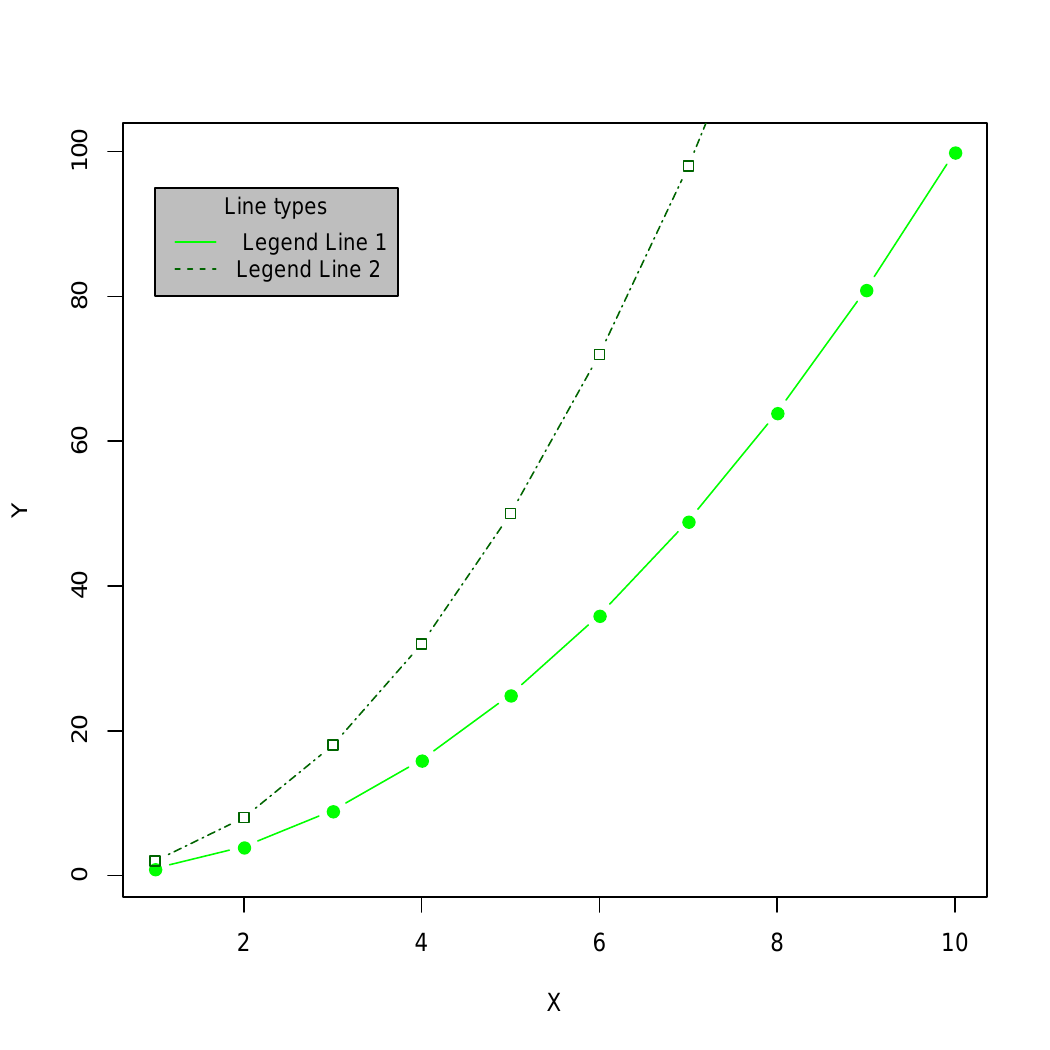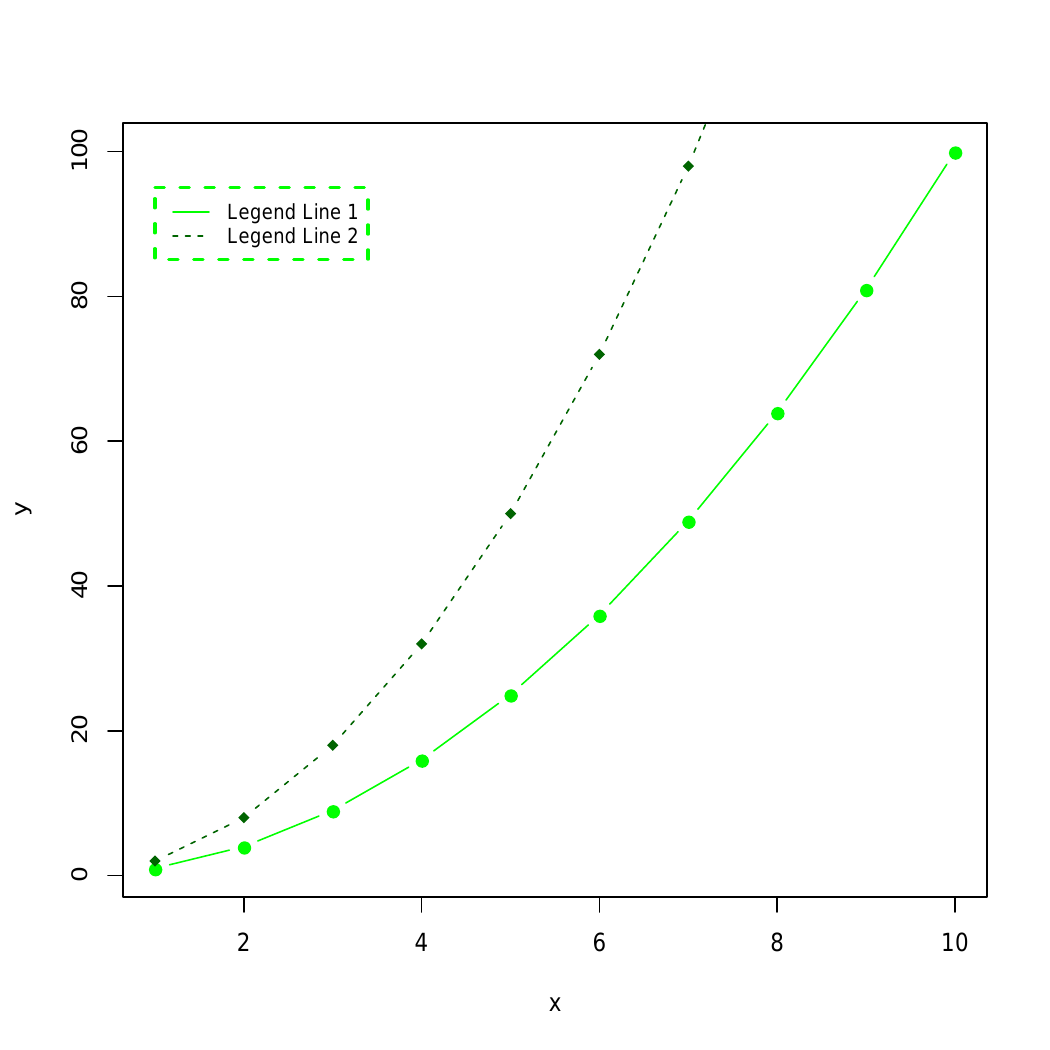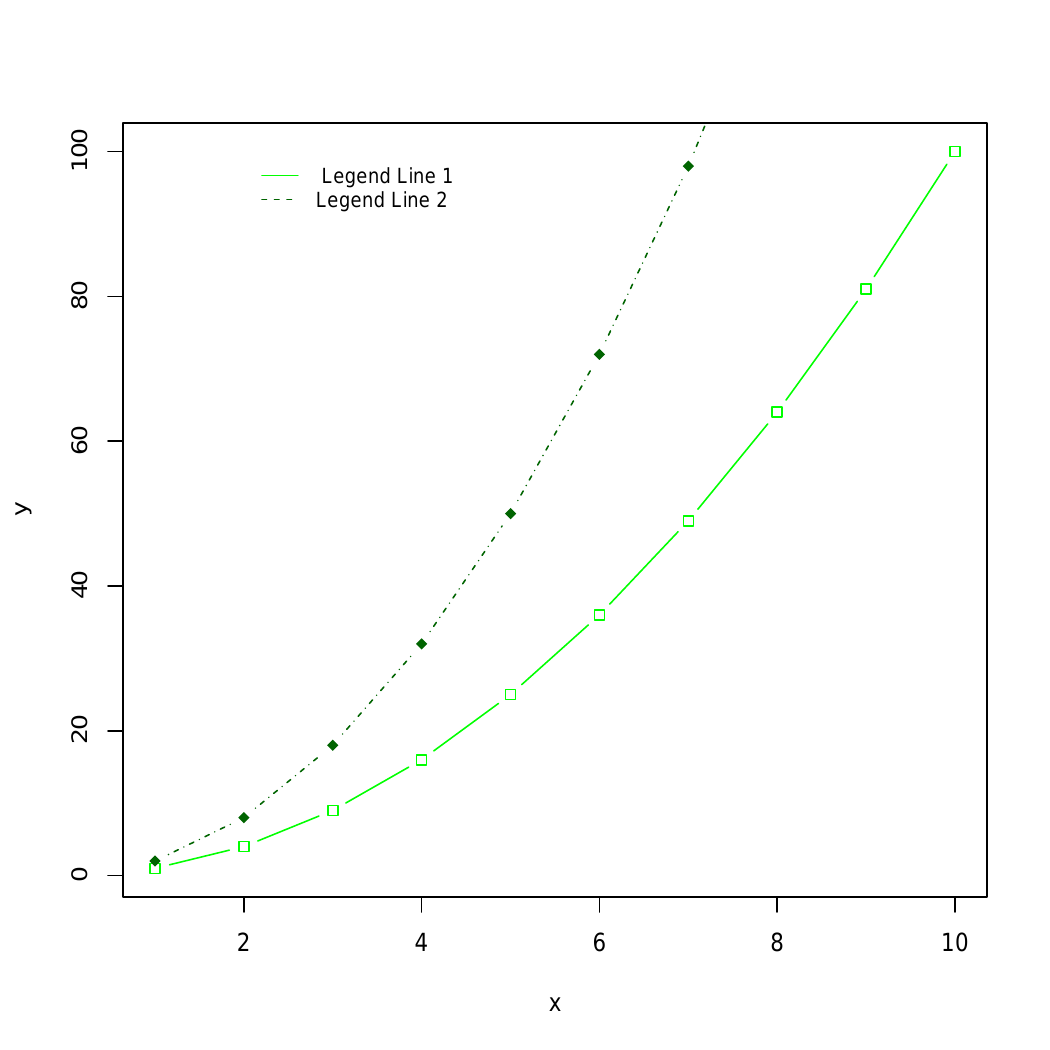# Describe Parts of a Chart in Graphical Form in R Programming – legend() Function

`legend()` function in R Language is used to add legends to an existing Plot. A legend is defined as an area of the graph plot describing each of the parts of the plot. The legend plot is used to show statistical data in graphical form.

Syntax:
legend(x, y, legend, fill, col, bg, lty, cex, title, text.font, bg)

Parameters:
x and y: These are co-ordinates to be used to position the legend
legend: Text of the legend
fill: Colors to use for filling the boxes of legend text
col: Colors of lines
bg: It defines background color for the legend box.
title: Legend title        (optional)
text.font: An integer specifying the font style of the legend (optional)

Returns: Legend plot

Example 1:

 `# Generate some data ` `x <- 1:8;  ` `y1 = x; y2 = 2 * y1 ` `plot``(x, y1, type = ``"b"``, pch = 22,  ` `     ``col = ``"green"``,  ` `     ``xlab = ``"X"``, ylab = ``"Y"``) ` `  `  `# Add a line ` `lines``(x, y2, pch = 18, col = ``"darkgreen"``, ` `           ``type = ``"b"``, lty = 2) ` `  `  `# Add a legend ` `legend``(1, 50, legend = ``c``(``"Legend Line 1"``, ``"Legend Line 2"``), ` `       ``col = ``c``(``"green"``, ``"darkgreen"``), lty = 2:3, cex = 0.6) `

Output:Example 2: Adding Title, text font and background color of the legend box

 `makePlot<-``function``(){ ` `  ``x<-1:10;  ` `  ``y1 = x * x; y2 = 2 * y1 ` `  ``plot``(x, y1, type = ``"b"``, pch = 19,  ` `                     ``col = ``"green"``,  ` `            ``xlab = ``"X"``, ylab = ``"Y"``) ` `  ``lines``(x, y2, pch = 22, col = ``"darkgreen"``,  ` `             ``type = ``"b"``, lty = 6) ` `} ` `makePlot``() ` `  `  `# Add a legend to the plot ` `legend``(1, 95, legend = ``c``(``" Legend Line 1"``, ``"Legend Line 2"``), ` `       ``col = ``c``(``"green"``, ``"darkgreen"``), lty = 1:2, cex = 0.9, ` `       ``title = ``"Line types"``, text.font = 6, bg = ``'gray'``) `

Output:Here, the `legend()` function is used to add legend to the plot and makePlot() function is used to manipulate font, background color.

Example 3: Another example to create border of the legend box

Syntax:
legendx, y, fill, col, bg, lty, cex=0.8, box.lty, box.lwd, box.col)

Parameters:
box.lty, box.lwd and box.col: line type, width and color for the legend box border, respectively.

 `makePlot<-``function``(){ ` `  ``x<-1:10;  ` `  ``y1 = x * x; y2 = 2 * y1 ` `  ``plot``(x, y1, type = ``"b"``, pch = 22,  ` `                     ``col = ``"green"``,  ` `             ``xlab = ``"x"``, ylab = ``"y"``) ` `   `  `  ``lines``(x, y2, pch = 18, col = ``"darkgreen"``,  ` `             ``type = ``"b"``, lty = 4) ` `} ` `  `  `# Change the border ` `makePlot``() ` `legend``(1, 100, legend = ``c``(``"Legend Line 1"``, ``"Legend Line 2"``), ` `       ``col = ``c``(``"green"``, ``"darkgreen"``), lty = 1:2, cex = 0.8, ` `       ``box.lty = 4, box.lwd = 2, box.col = ``"green"``) `

Output:Example 4: Below is illustration to remove legend border by using box.lty = 0 in legend() function

Syntax:
legendx, y, fill, col, bg, lty, cex=0.8, box.lty=0)

Parameter:
box.lty: Box line width

 `makePlot<-``function``(){ ` `  ``x<-1:10;  ` `  ``y1 = x * x; y2 = 2 * y1 ` `  ``plot``(x, y1, type = ``"b"``, pch = 22,  ` `                     ``col = ``"green"``,  ` `            ``xlab = ``"x"``, ylab = ``"y"``) ` `   `  `  ``lines``(x, y2, pch = 18, col = ``"darkgreen"``,  ` `             ``type = ``"b"``, lty = 4) ` `} ` `  `  `# Remove legend border using box.lty = 0 ` `makePlot``() ` `legend``(2, 100, legend = ``c``(``"Legend Line 1"``,  ` `                          ``"Legend Line 2"``), ` `       ``col = ``c``(``"green"``, ``"darkgreen"``),  ` `       ``lty = 1:2, cex = 0.8, box.lty = 0) `

Output:In example 3 and example 4 box.lty, box.lwd and box.col can be used to modify the line type, width, and color for the legend box border, respectively are used to modify the arguments.

My Personal Notes arrow_drop_upCheck out this Author's contributed articles.

If you like GeeksforGeeks and would like to contribute, you can also write an article using contribute.geeksforgeeks.org or mail your article to contribute@geeksforgeeks.org. See your article appearing on the GeeksforGeeks main page and help other Geeks.

Please Improve this article if you find anything incorrect by clicking on the "Improve Article" button below.

Article Tags :

Be the First to upvote.

Please write to us at contribute@geeksforgeeks.org to report any issue with the above content.